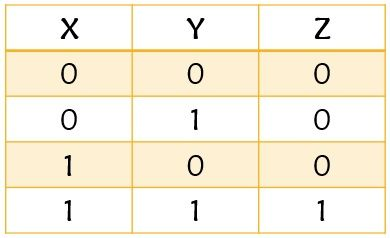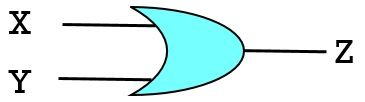# Difference Between AND Gate and OR Gate

Both AND logic gate and OR logic gate are the two basic logic gates used in digital circuits. However, the two are mainly differentiated on the basis of logic operation performed by them. The crucial difference between AND gate and OR gate is that AND gate performs multiplication of the digital inputs. As against the OR logic gate is used to execute the addition of the digital inputs.

It is known that logic gates are basically defined as the electronic circuits that are used to implement Boolean functions. The digital logic gates serve as the basic building block of electronic systems. As digital logic designs permit only two states or voltage levels that are generally expressed as 1 or 0 and sometimes are referred as either high low or true false respectively.

Basically, there are various operations performed by different logic gates that include addition, multiplication, inverse, etc. These operations distinct the different logic gates. The operation performed by the logic gates is referred as a boolean expression. Here in this article, we will discuss the factors that differentiate an AND gate from an OR gate.

## Comparison Chart

Basis for ComparisonAND GateOR Gate
BasicIt executes logical multiplication.OR gate executes logical summation.
SymbolRuleLogical conjunctionLogical disjunction
Boolean ExpressionX . YX + Y
OutputLogic high output is achieved when necessarily both the inputs are high.Logic high is achievable in case of even single high input.

### Definition of AND gate

A logic gate that is designed to execute the logical multiplication of binary inputs is known as AND gate. We know the basic product rule that if 1 and 0 is multiplied then automatically the resultant will be 0. However, when 1 and 1 are multiplied then its product will be 1 itself.

This rule is followed by AND logic gate in the digital system.The symbolic representation of the AND gate is shown above. Here we have considered X and Y as the two inputs of the AND logic gate and Z to be the output.

The figure below represents the truth table of AND gate:As we can see in the truth table representation that when both X and Y are logic low i.e., 0 then the value of Z is also logic low. Even in the case when any one of the given input is high then also the value of output will be low. But when both the digital values at the input of the AND logic gate is high then the gate produces a logic high signal at its output.

Here we have discussed the logic operation for two inputs but it can be expanded to more than 2 inputs.

### Definition of OR gate

A type of logic gate that is designed to perform logical addition of two applied inputs is known as the OR gate. This logic gate follows the basic rule of the addition of digital inputs. The basic rule of summation is such that even when a single input is one then the output will also be 1. However, in the case of both the inputs as 0, the output will automatically become 0.

Let us see the symbolic representation of the OR logic gate:Here X and Y represent the two inputs of the OR logic gate. While Z is the output. The truth table representation of OR logic gate:It is clear from the truth table representation that when both the inputs are 0, then the output will also be 0. However, when any one or both the inputs become logic high then the output will also be high. This is the reason why the tabular representation shows the output to be high when even a single input is high. While the output automatically turns out to be 0 when only even a single input is not 1.

## Key Differences Between AND Gate and OR Gate

1. AND gate provides the product of two binary values as its output. However, the OR gate gives the summation of the two applied binary inputs as its output.
2. AND gate follows the rule of logical conjunction. As against OR gate follows logical disjunction.
3. The boolean expression of the AND gate is represented as A.B. While the boolean expression of the OR gate is given as A+B, where A and B are considered to be the two applied inputs.
4. In AND gate, logic high output is achieved only when both the applied inputs are high. While in the OR gate, a logic high is achieved if either anyone or both the inputs are high.

### Conclusion

So, we can conclude that both AND and OR are the two basic logic gates designed to execute boolean operations. However, the crucial difference lies in the operation performed by them.